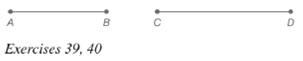Chapter 7.1, Problem 39EElementary Geometry For College St...

7th Edition
Alexander + 2 others
ISBN: 9781337614085

Solutions

Chapter
SectionElementary Geometry For College St...

7th Edition
Alexander + 2 others
ISBN: 9781337614085
Textbook Problem

In Exercises 39 and 42, refer to the line segments shown.Construct an isosceles right triangle that has hypotenuse A B - .To determine

To construct:

The isosceles triangle that has hypotenuse AB-.

Explanation

Procedure:

An angle inscribed in a semicircle is a right angle triangle.

Construct a circle whose diameter is the midpoint M of the hypotenuse and whose radius equals one-half of the length of the hypotenuse.

1. Construct a line segment AB-.

2. Locate the midpoint M.

3. Draw a line MC perpendicular to the line AB-.

3. Take M as center and MA or MB as radius draw a circle and locate the point C

Still sussing out bartleby?

Check out a sample textbook solution.

See a sample solution

The Solution to Your Study Problems

Bartleby provides explanations to thousands of textbook problems written by our experts, many with advanced degrees!

Get Started

Find more solutions based on key concepts